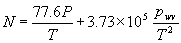## Raman scattering

When a photon interacts with a molecule, such as happens when the laser radiation from a lidar scatters from atmospheric molecules, it can gain or lose energy as a result of transitions within the molecule. This is known as Raman scattering, which is an inelastic quantum-mechanical scattering process. It results in a change in wavelength of the radiation, and this change is a characteristic of the scattering molecule. This makes it possible to select radiation from different molecules. This scattering process is much weaker than the simple elastic process in which there is no change of wavelength. Raman scattering is used in many applications other than lidar, such as the analysis of chemicals in laboratory work.

## Water vapour measurement

The water vapour mixing ratio (the proportion of water molecules by either number or mass) is measured from the ratio of the Raman scattering signal from water vapour to that from nitrogen. The proportion of nitrogen in the atmosphere is close to constant as a function of height over the range of interest in these measurements. Both of the transitions used for the measurement are from the first vibrational level of the molecule to the ground state. For our transmitted wavelength of 354.7nm, the water vapour and nitrogen lines are at 407.8 and 386.7nm respectively.

## Temperature measurement

At a temperature T, the fraction of molecules which are in an excited state of energy E is proportional to the factor exp (-E/kT). This factor is small for the first excited vibrational state (as used in water vapour measurements), but is large for the first few rotational states, which are much more closely spaced in energy. The distribution of molecules between these energy states is a function of temperature, so by measuring Raman signals corresponding to two different sets of rotational states it is possible to determine the temperature of the scattering molecules. In our system we use filters centred at 353.0 and 353.9nm. These select scattering from groups of lines from both nitrogen and oxygen molecules.